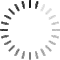# C++ implicit ans explicit Typecasting

0
0

Hello sir,

The question is regarding c++ class Hierarchy Audio 10. I have tried a small program to print the address of multiple class hierarchy below is the snapshot.

class A
{
public:
A() :a(1), b(2), c(3) {}
int a;
int b;
int c;
};
class B
{
public:
B() :a(10), b(20), c(30) {}
int a;
int b;
int c;
};
class C :public A, public B
{
public:
C() :a(100), b(200), c(300) {}
int a;
int b;
int c;
};

{
std::cout << “funcA :” << addr << std::endl;
std::cout << “funcA A* data:” << addr->c << std::endl; //compiler doing implicit typecasting incase &c
std::cout << “funcA C* data:” << ((C*)addr)->c << std::endl << std::endl;

std::cout << std::endl;
}

{
std::cout << “funcB :” << addr << std::endl;
std::cout << “funcB C*:” << (C*)addr << std::endl;
std::cout << “funcB B* data:” << addr->c << std::endl;
std::cout << “funcB C* data:” << ((C*)addr)->c << std::endl << std::endl;

}

{
std::cout << “funcC C*:” << addr << std::endl;
std::cout << “funcC A*:” << (A*)addr << std::endl;
std::cout << “funcC B*:” << (B*)addr << std::endl <;

std::cout << “funcC C* data” << addr->c << std::endl;
}

int main()
{

A a;
B b;
C c;

std::cout << &c << std::endl;
funcC(&c); //Invocation 1
funcA(&c); // Invocation 2
funcB(&c); // Invocation 3

return 0;
}

output >>

0078F8EC
funcC C*:0078F8EC
funcC A*:0078F8EC
funcC B*:0078F8F8
funcC C* data 300

funcA :0078F8EC
funcA A* data:3
funcA C* data:300

funcB :0078F8F8
funcB C*:0078F8EC
funcB B* data:30
funcB C* data:300

In invocation 1 on object c , both A* and C* are pointing to a same address, how does compiler interprets internally to invoke C’s subobject data from Composite object.

In invocation 2 on object c, though both A * and C * holding same address , How does typecasting addr to (C*), able to print C’s subobject data.

In invocation 3 I see the delta change of 12 byte is added  while doing implicit typecasting t0 B* , and again doing explicit typecasting t0 C * having the same address as A * how is compiler is able to print C’s data.

How compiler knows exactly to which member of composite object to invoke, when explicit typecasting is done.

Regards

G V Siddesh

• You must to post comments
0
0

Dear Students,  for your sample classes you have defined, why don’t you write down object images as indicated in Sessions 12, 13 in implementation of classes and you can immediately guess how compiler is getting the offsets of various members of sub-objects.

MLS Shastry

• You must to post comments
Showing 1 result